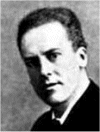Go to content
The following calculations are needed when finding Pearson's product moment correlation coefficient or pmcc:
S_{xx} = \displaystyle \sum x^2 - \frac{(\sum x)^2}{n}
S_{yy} = \displaystyle \sum y^2 - \frac{(\sum y)^2}{n}
S_{xy} = \displaystyle \sum xy - \frac{(\sum x)(\sum y)}{n}

## Summary/Background

The pmcc can be found using:
r = \displaystyle \frac{S_{xy} }{\sqrt{S_{xx} S_{yy} } }Karl Pearson FRS (27 March 1857 – 27 April 1936) established the discipline of mathematical statistics. A sesquicentenary conference was held in London on 23 March 2007, to celebrate the 150th anniversary of his birth.
In 1911 he founded the world's first university statistics department at University College London. He was a proponent of eugenics, and a protege and biographer of Sir Francis Galton. He was also a socialist. Pearson was instrumental in the development of regression and correlation theory. One of his classic data sets (originally collected by Galton) involves the regression of sons' height upon that of their fathers'. Pearson built a 3-dimensional model of this data set (which remains in the care of the Statistical Science Department) to illustrate the ideas. The Pearson product-moment correlation coefficient is named after him, and it was the first important "effect size" to be introduced into statistics.You can get a better display of the maths by downloading special TeX fonts from jsMath. In the meantime, we will do the best we can with the fonts you have, but it may not be pretty and some equations may not be rendered correctly.

## Glossary

### coefficient

The constant value in an expression, for example in 3x the coefficient of x is 3.

### correlation

The degree of relationship between two random variables, in the range -1 to 1.

### moment

The turning effect of a force whose value depends on the magnitude of the force and where it is applied.

### pmcc

The product moment correlation coefficient

### product moment correlation coefficient

Often abbreviated to PMCC; a measure of correlation.

### regression

A statistical model, whereby the expected value of a variable y is expressed in terms of known values of x.

### union

The union of two sets A and B is the set containing all the elements of A and B.

Full Glossary List

## This question appears in the following syllabi:

SyllabusModuleSectionTopicExam Year
AQA A-Level (UK - Pre-2017)S1Correlation and RegressionCorrelation-
AQA A2 Maths 2017StatisticsCorrelation and RegressionProduct Moment Correlation Coefficient-
AQA AS Further Maths 2017StatisticsExtraProduct Moment Correlation Coefficient-
AQA AS/A2 Further Maths 2017StatisticsExtraProduct Moment Correlation Coefficient-
AQA AS/A2 Maths 2017StatisticsCorrelation and RegressionProduct Moment Correlation Coefficient-
CCEA A-Level (NI)S2Correlation and RegressionCorrelation-
Edexcel A-Level (UK - Pre-2017)S1Correlation and RegressionCorrelation-
Edexcel A2 Maths 2017StatisticsRegression and CorrelationProduct Moment Correlation Coefficient-
Edexcel AS Further Maths 2017Further Statistics 2CorrelationProduct Moment Correlation Coefficient-
Edexcel AS/A2 Further Maths 2017Further Statistics 2CorrelationProduct Moment Correlation Coefficient-
Edexcel AS/A2 Maths 2017StatisticsRegression and CorrelationProduct Moment Correlation Coefficient-
I.B. Higher Level7Correlation and RegressionCorrelation-
OCR A-Level (UK - Pre-2017)S1Correlation and RegressionCorrelation-
OCR A2 Maths 2017StatisticsUsing and Testing Correlation CoefficientProduct Moment Correlation Coefficient-
OCR AS Further Maths 2017StatisticsCorrelationProduct Moment Correlation Coefficient-
OCR MEI A2 Maths 2017StatisticsUsing and Testing Correlation CoefficientProduct Moment Correlation Coefficient-
OCR MEI AS Further Maths 2017Statistics ACorrelationProduct Moment Correlation Coefficient-
OCR-MEI A-Level (UK - Pre-2017)S2Correlation and RegressionCorrelation-
Pre-U A-Level (UK)ProbCorrelation and RegressionCorrelation-
Scottish (Highers + Advanced)HSCorrelation and RegressionCorrelation-